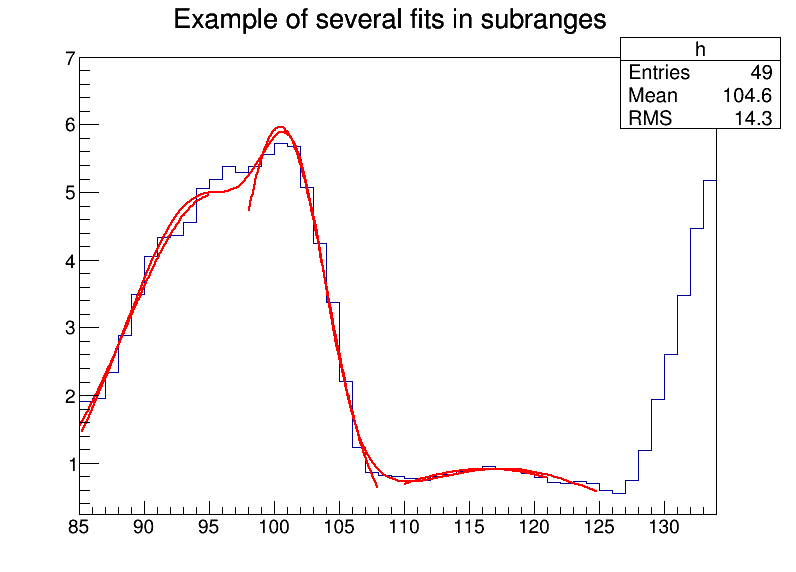In :
import rootnotes


An histogram is created and filled with the bin contents and errors defined in the table below.

3 gaussians are fitted in sub-ranges of this histogram.

A new function (a sum of 3 gaussians) is fitted on another subrange

Note that when fitting simple functions, such as gaussians, the initial values of parameters are automatically computed by ROOT.

In the more complicated case of the sum of 3 gaussians, the initial values of parameters must be given. In this particular case, the initial values are taken from the result of the individual fits.

In :
from ROOT import TH1F, TF1
from array import array

In :
gROOT.Reset()
canvas = rootnotes.default_canvas()

In :
x = ( 1.913521, 1.953769, 2.347435, 2.883654, 3.493567,
4.047560, 4.337210, 4.364347, 4.563004, 5.054247,
5.194183, 5.380521, 5.303213, 5.384578, 5.563983,
5.728500, 5.685752, 5.080029, 4.251809, 3.372246,
2.207432, 1.227541, 0.8597788,0.8220503,0.8046592,
0.7684097,0.7469761,0.8019787,0.8362375,0.8744895,
0.9143721,0.9462768,0.9285364,0.8954604,0.8410891,
0.7853871,0.7100883,0.6938808,0.7363682,0.7032954,
0.6029015,0.5600163,0.7477068,1.188785, 1.938228,
2.602717, 3.472962, 4.465014, 5.177035 )

In :
np = len(x)
h = TH1F( 'h', 'Example of several fits in subranges', np, 85, 134 )
h.SetMaximum( 7 )

for i in xrange(np):
h.SetBinContent( i+1, x[i] )

par = array( 'd', 9*[0.] )
g1 = TF1( 'g1', 'gaus',  85,  95 )
g2 = TF1( 'g2', 'gaus',  98, 108 )
g3 = TF1( 'g3', 'gaus', 110, 121 )

total = TF1( 'total', 'gaus(0)+gaus(3)+gaus(6)', 85, 125 )
total.SetLineColor( 2 )
h.Fit( g1, 'R' )
h.Fit( g2, 'R+' )
h.Fit( g3, 'R+' )

par1 = g1.GetParameters()
par2 = g2.GetParameters()
par3 = g3.GetParameters()

par, par, par = par1, par1, par1
par, par, par = par2, par2, par2
par, par, par = par3, par3, par3

total.SetParameters( par )
fit = h.Fit( total, 'R+' )

In :
canvas

Out:In :
from sympy import init_printing; init_printing()

In :
from sympy import *

In :
x = Symbol("x")
k = Symbol("k")
y = Symbol("y")

b = Symbol("b")

t = sin(2*pi*x*(k**2)) / (4*(pi**2)*(k**5)) + (x**2) / (2*k)

t

Out:
$$\frac{x^{2}}{2 k} + \frac{1}{4 \pi^{2} k^{5}} \sin{\left (2 \pi k^{2} x \right )}$$
In :
t.evalf(subs={'x':1, 'k':2})

Out:
$$0.25$$

Courtesy of MathJax, you can include mathematical expressions both inline:

$e^{i\\pi} + 1 = 0$ and displayed

$$e^x=\sum_{i=0}^\infty \frac{1}{i!} x^i$$
$$c = \sqrt{a^2 + b^2}$$
In :
from IPython.display import display, Math, Latex
display(Math(r'F(k) = \int_{-\infty}^{\infty} f(x) e^{2\pi i k} dx'))

$$F(k) = \int_{-\infty}^{\infty} f(x) e^{2\pi i k} dx$$
In :
%%latex
\begin{aligned}
\nabla \times \vec{\mathbf{B}} -\, \frac1c\, \frac{\partial\vec{\mathbf{E}}}{\partial t} & = \frac{4\pi}{c}\vec{\mathbf{j}} \\
\nabla \cdot \vec{\mathbf{E}} & = 4 \pi \rho \\
\nabla \times \vec{\mathbf{E}}\, +\, \frac1c\, \frac{\partial\vec{\mathbf{B}}}{\partial t} & = \vec{\mathbf{0}} \\
\nabla \cdot \vec{\mathbf{B}} & = 0
\end{aligned}

\begin{aligned} \nabla \times \vec{\mathbf{B}} -\, \frac1c\, \frac{\partial\vec{\mathbf{E}}}{\partial t} & = \frac{4\pi}{c}\vec{\mathbf{j}} \\ \nabla \cdot \vec{\mathbf{E}} & = 4 \pi \rho \\ \nabla \times \vec{\mathbf{E}}\, +\, \frac1c\, \frac{\partial\vec{\mathbf{B}}}{\partial t} & = \vec{\mathbf{0}} \\ \nabla \cdot \vec{\mathbf{B}} & = 0 \end{aligned}